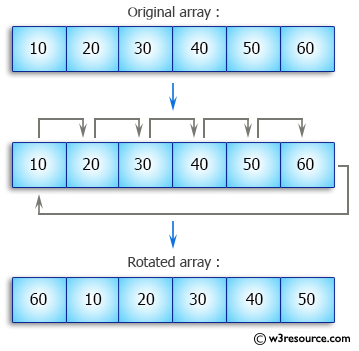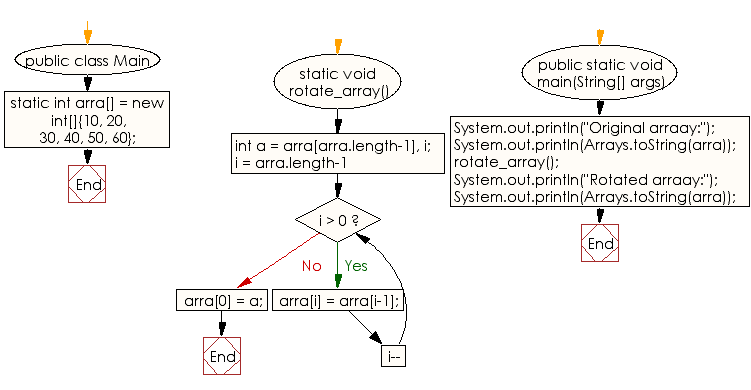﻿ Java: Cyclically rotate a given array clockwise by one# Java Array Exercises: Cyclically rotate a given array clockwise by one

## Java Array: Exercise-45 with Solution

Write a Java program to cyclically rotate a given array clockwise by one.

Pictorial Presentation:Sample Solution:

Java Code:

``````import java.util.Arrays;
public class Main
{
static int arra[] = new int[]{10, 20, 30, 40, 50, 60};

static void rotate_array()
{
int a = arra[arra.length-1], i;
for (i = arra.length-1; i > 0; i--)
arra[i] = arra[i-1];
arra = a;
}

public static void main(String[] args)
{
System.out.println("Original arraay:");
System.out.println(Arrays.toString(arra));

rotate_array();

System.out.println("Rotated arraay:");
System.out.println(Arrays.toString(arra));
}
}
```
```

Sample Output:

```
Original arraay:
[10, 20, 30, 40, 50, 60]
Rotated arraay:
[60, 10, 20, 30, 40, 50]
```

Flowchart:Visualize Java code execution (Python Tutor):

Java Code Editor:

Improve this sample solution and post your code through Disqus

What is the difficulty level of this exercise?

Test your Programming skills with w3resource's quiz.

﻿

## Java: Tips of the Day

countOccurrences

Counts the occurrences of a value in an array.

Use Arrays.stream().filter().count() to count total number of values that equals the specified value.

```public static long countOccurrences(int[] numbers, int value) {
return Arrays.stream(numbers)
.filter(number -> number == value)
.count();
}
```

Ref: https://bit.ly/3kCAgLb